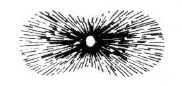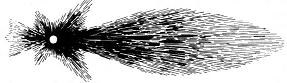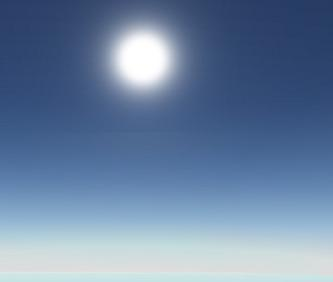以前由于硬件限制，很多游戏的天空和地面颜色主要是用贴图模拟，近来硬件的发展，越来越多的游戏开始采用基于比较真实的大气散射模型来实时计算。很多文章的计算最终都将眼睛高度和角度作为参数，这里主要按照Sean O’Neil系列的方法来(其实它也是Nishita的改进)。

原理可以简单归结为：光从大气外圈，散射之后进入眼睛。散射本身是一种衰减行为，由于星球通常离太阳比较远，所以我们近似认为在大气的"顶端"光照相等，这是一个重要的近似，让相机在任何地方的时候，都可以用同样的方式来计算，所以只要是往地面的路径，则散射增加，只要是玩天空的路径，则散射减少。

Iv(λ) = Is(λ) * K(λ) * F(θ，g) * PbPa(exp(-b/H0) * exp(-t(PPc, λ) – t(PPa, λ)))ds;Rayleigh散射的近似能量分布F(θ，g) = (3 * (1 – g2) * (1 + cos2θ)) / (2 * (2 + g2) * (1 + g2 – 2 * g * cosθ))3/2;

亮度公式中的积分部分

PbPa(exp(-b/H0) * exp(-t(PPc, λ) – t(PPa, λ)))ds;

t(PaPb, λ) = 4 * π * K(λ) * PbPaexp(-b/H0)ds;

PaPb分别为观察点和太阳离计算的采样点最近的大气位置，如果观察点在大气中则Pa就是观察点的位置。公式中的积分称为空气的视觉深度(optical depth)，以前一般用预计算查找表来进行，这样不利于在GPU计算，gpugems2Sean O’Neil通过图形曲线发现在观察角度固定的时候，每个高度上(01)的积分值可以用地面的值(高度为0时候的值)乘于exp(-b/H0)来近似表示，但对于不同角度的缩放量曲线是一条类似指数函数的曲线，没有很好的表示方式，Sean O’Neil自己根据图形曲线用了一条逼近的公式来计算

Scale(ξ) = H0 * exp(-0.00287 + (1 - cos *ξ)(0.459 + (1 - cos *ξ)

(3.83 + (1 - cos *ξ)(-6.80 +(1 - cos *ξ)5.25))));

这样全部问题就可以放到GPU中解决了，对于每个顶点首先从camera到该点(PaPb)做一些采样(次数越多越能逼近，但计算量也越大)，然后对每个采样点根据角度计算Scale(ξ)，再根据高度计算optical depth以及t(PaPb, λ)，然后根据各段采样长度就可以计算最终的强度。

地面颜色的计算与此类似，不过增加了一次与原颜色的混合。

Ig(λ) = Iv(λ) + Isrc(λ) * exp(-t(PaPb, λ));